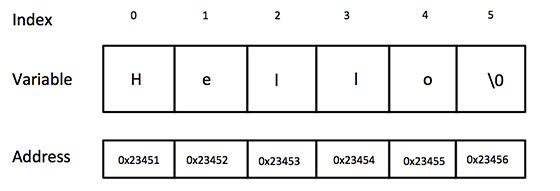# # String in C

You will be able to :

Define string

Declaration of String

Initialization of String

Use string handling function

## # String

In C programming, array of character are called strings. A string is terminated by null character /0.

Array is the collection of similar data under common name.

A common name is the name of string or array.

For example:

``````char stringname  = "Hello";
``````
1

Here stringname is common name .We will study String Decleration below Section.

C compiler represent above string as below image (string representation)## # Declaration of String

It is declared as:

``````char string_name[size];

E.g: char name;
``````
1
2
3

Strings can also be declared using pointer.

``````char *p
``````
1

## # Initialization of String

It can be initialized when they are declared

``````char c[]="abcd";
OR,

char c="abcd";

OR,

char c[]={'a','b','c','d','\0'};

OR;

char c={'a','b','c','d','\0'}; .

``````
1
2
3
4
5
6
7
8
9
10
11
12
13

String can also be initialized using pointers

``````char *c="abcd";
``````
1

## # String Handling Function

There are different libraries or built-in function in C for string manipulation.These function are defined in header file <string.h>

All string manipulation can be done manually by the programmer but, this makes programming complex and large.

To solve this, the C supports a large number of string handling functions.

``````strlen()  -  Calculates the length of string

strcpy() -   Copies a string to another string

strcat()  -  Concatenates(joins) two strings

strcmp() -   Compares two string

strlwr()  -  Converts string to lowercase

strupr()  -  Converts string to uppercase
``````

### # 1. strlen():

This is used to return the length of string

It returns the number of characters in the string without including null character

Syntax:

``````integer_variable = strlen(string);
``````
1

### # 2. strcpy() :

It copies one string to another

Syntax:

``````strcpy(destination_string , source_string);
``````
1

e.g strcpy(a,b) i.e the content of b is copied to a

### # 3. strcat():

It joins to strings together

It stores the content of second string at the end of the first one.

``````E.g: strcat (string1 , string2  );

i.e string1 = string1 + string2;
``````
1
2
3

### # 4. strcmp():

It compares two string

It takes two strings as parameters and returns the integer value.

``````  0 if both string are same

less than zero if  the first string is less than the second one.

Greater than zero if the first string is more than the second
``````

## # 5. strrev () :

It is used to reverse all character of string except the null character

Syntax:

``````    strrev ( string );

E.g strrev(str) i.e it reverses the characters in string str and stores the reversed string in the str
``````
1
2
3